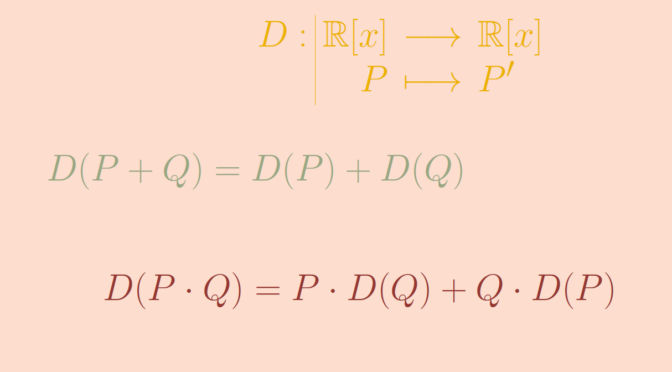# Group homomorphism versus ring homomorphism

A ring homomorphism is a function between two rings which respects the structure. Let’s provide examples of functions between rings which respect the addition or the multiplication but not both.

### An additive group homomorphism that is not a ring homomorphism

We consider the ring $$\mathbb R[x]$$ of real polynomials and the derivation $\begin{array}{l|rcl} D : & \mathbb R[x] & \longrightarrow & \mathbb R[x] \\ & P & \longmapsto & P^\prime \end{array}$ $$D$$ is an additive homomorphism as for all $$P,Q \in \mathbb R[x]$$ we have $$D(P+Q) = D(P) + D(Q)$$. However, $$D$$ does not respect the multiplication as $D(x^2) = 2x \neq 1 = D(x) \cdot D(x).$ More generally, $$D$$ satisfies the Leibniz rule $D(P \cdot Q) = P \cdot D(Q) + Q \cdot D(P).$

### A multiplication group homomorphism that is not a ring homomorphism

The function $\begin{array}{l|rcl} f : & \mathbb R & \longrightarrow & \mathbb R \\ & x & \longmapsto & x^2 \end{array}$ is a multiplicative group homomorphism of the group $$(\mathbb R, \cdot)$$. However $$f$$ does not respect the addition.